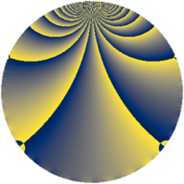# Properties

 Label 1840.2.tLevel $1840$ Weight $2$ Character orbit 1840.t Rep. character $\chi_{1840}(1243,\cdot)$ Character field $\Q(\zeta_{4})$ Dimension $528$ Sturm bound $576$

# Related objects

## Defining parameters

 Level: $$N$$ $$=$$ $$1840 = 2^{4} \cdot 5 \cdot 23$$ Weight: $$k$$ $$=$$ $$2$$ Character orbit: $$[\chi]$$ $$=$$ 1840.t (of order $$4$$ and degree $$2$$) Character conductor: $$\operatorname{cond}(\chi)$$ $$=$$ $$80$$ Character field: $$\Q(i)$$ Sturm bound: $$576$$

## Dimensions

The following table gives the dimensions of various subspaces of $$M_{2}(1840, [\chi])$$.

Total New Old
Modular forms 584 528 56
Cusp forms 568 528 40
Eisenstein series 16 0 16

## Trace form

 $$528q + 12q^{8} - 528q^{9} + O(q^{10})$$ $$528q + 12q^{8} - 528q^{9} - 16q^{12} - 28q^{18} - 12q^{20} - 16q^{22} + 32q^{26} + 12q^{28} - 56q^{30} + 40q^{32} + 16q^{34} - 24q^{35} - 68q^{38} + 28q^{40} - 40q^{42} + 64q^{43} - 32q^{44} + 48q^{47} - 28q^{48} - 28q^{50} - 16q^{52} + 32q^{54} + 76q^{58} + 32q^{59} + 40q^{60} - 60q^{62} - 16q^{66} - 48q^{67} - 16q^{68} + 16q^{70} - 64q^{71} + 40q^{72} - 16q^{73} - 56q^{74} - 88q^{75} + 124q^{78} + 12q^{80} + 528q^{81} + 76q^{82} + 120q^{84} + 16q^{86} - 112q^{87} + 156q^{88} + 164q^{90} - 32q^{91} - 48q^{94} + 104q^{96} - 48q^{98} + 64q^{99} + O(q^{100})$$

## Decomposition of $$S_{2}^{\mathrm{new}}(1840, [\chi])$$ into newform subspaces

The newforms in this space have not yet been added to the LMFDB.

## Decomposition of $$S_{2}^{\mathrm{old}}(1840, [\chi])$$ into lower level spaces

$$S_{2}^{\mathrm{old}}(1840, [\chi]) \cong$$ $$S_{2}^{\mathrm{new}}(80, [\chi])$$$$^{\oplus 2}$$